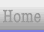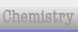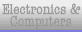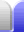# Factorising Numbers

Factorisation is a complicated sounding word that simply means finding the individual numbers that multiply together to give a larger number. These individual numbers are called factors. For example, 6 and 8 are factors of 48 because 6 x 8 = 48.

However, we can sometimes factorise these factors, as is the case with both 6 and 8. If we cannot factorise any further we have found the prime factors. The prime factors are prime numbers, since they won't have factors themselves. (A prime number is a number which is only divisible by itself and 1.)

This can probably be most easily seen with a few examples. Say we have a number 6. We note that it is an even number, so we know that 2 will be one of the factors.

6 = 2 x 3

Both 2 and 3 are prime numbers, so we can't factorise 6 any further. Thus we can say that the prime factors of 6 are 2 and 3.

Let's have a crack at a bigger number, say 10,080. Straight away we see that it's even, so 2 is one of the factors. The next numbers we get are also even, so another factor is 2.

 10,080 = 2 x 5,040 = 2 x 2 x 2,520 = 2 x 2 x 2 x 1,260 = 2 x 2 x 2 x 2 x 630 = 2 x 2 x 2 x 2 x 2 x 315

At this point we know that there aren't any more 2s, since we're down to an odd number. It's pretty obvious that another factor is 5, so let's do that. (It doesn't matter at all what order we find them in.)

 10,080 = 2 x 2 x 2 x 2 x 2 x 5 x 63

And with that we're down to a number that we should recognise from our times tables.

 10,080 = 2 x 2 x 2 x 2 x 2 x 5 x 7 x 9 = 2 x 2 x 2 x 2 x 2 x 5 x 7 x 3 x 3 = 2 x 2 x 2 x 2 x 2 x 3 x 3 x 5 x 7

That last line was just rearranging the factors to put the 3s in a tidier place. So the prime factors of 10,080 are 2, 2, 2, 2, 2, 3, 3, 5, and 7. This is a slightly long-winded way of writing the factors, so if we want we can use exponents.

10,080 = 25 x 32 x 5 x 7

There are some very handy Factorising Tricks we can use to make it easier to find factors, although sometimes those tricks will not be much use.

 1,234,567,890 = 10 x 123,456,789 (divisible by 9) = 2 x 5 x 9 x 13,717,421 (no simple tricks will work) = 2 x 3 x 3 x 5 x 3,607 x 3,803

And that's as far as we can go, because 3,607 and 3,803 are prime numbers. So the prime factors of 1,234,567,890 are 2, 3, 3, 5, 3607 and 3803.Home Astronomy Chemistry Electronics & Computers Mathematics Physics Field Trips Turn on javascript for email link